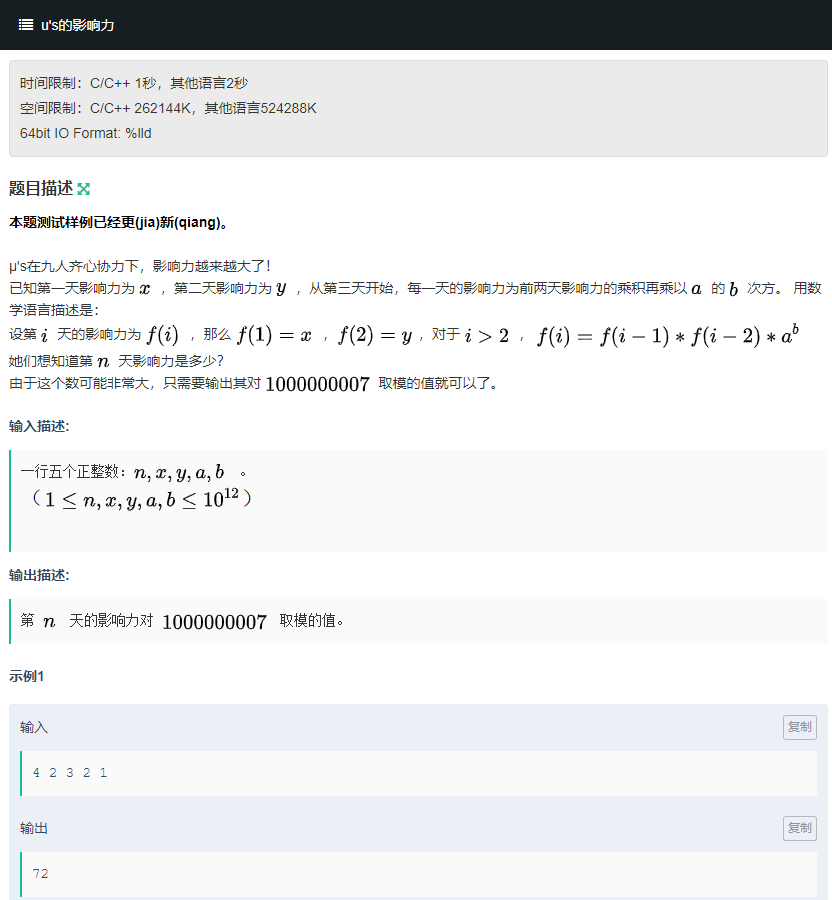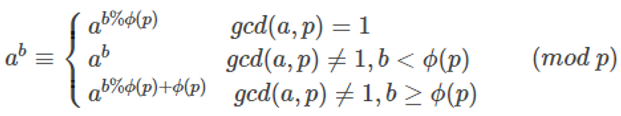## 题目传送门：https://ac.nowcoder.com/acm/contest/3002/J## 完整思路：

$$f(1)=x$$

$$f(2)=y$$

$$f(3)=x^1y^1(a^b)^1$$

$$f(4)=x^1y^2(a^b)^{2}$$

$$f(5)=x^2y^3(a^b)^{4}$$

$$f(6)=x^3y^5(a^b)^{7}$$

$$f(7)=x^5y^8(a^b)^{12}$$

$$f(n)=x^{k1}y^{k2}(a^b)^{k3}$$

$$F(1)=1，F(2)=1$$

$$F(n)=F(n-1)+F(n-2)（n>=3）$$

$$\begin{bmatrix} 1 & 1 \ 1 & 0 \ \end{bmatrix} * \begin{bmatrix} F(n-1) \ F(n-2) \ \end{bmatrix} = \begin{bmatrix} F(n) \ F(n-1) \ \end{bmatrix}$$

$$A* \begin{bmatrix} F(n-1) \ F(n-2) \ \end{bmatrix} = \begin{bmatrix} F(n) \ F(n-1) \ \end{bmatrix}，其中A=\begin{bmatrix} 1 & 1 \ 1 & 0 \ \end{bmatrix}$$

$$A^{n-2}* \begin{bmatrix} F(2) \ F(1) \ \end{bmatrix} = \begin{bmatrix} F(n) \ F(n-1) \ \end{bmatrix}(n>=2)，其中A=\begin{bmatrix} 1 & 1 \ 1 & 0 \ \end{bmatrix}$$

$$\begin{bmatrix}S_{00} & S_{01} \ S_{10} &S_{11} \ \end{bmatrix} * \begin{bmatrix} F(2) \ F(1) \ \end{bmatrix} = \begin{bmatrix} F(n) \ F(n-1) \ \end{bmatrix}(n>=2)，其中S=A^{n-2}$$

$$f(n)=x^{k1}y^{k2}(a^b)^{k3} \%p$$## AC代码：（4ms）

#include <bits/stdc++.h>
using namespace std;
typedef long long ll;
const ll p=1e9+7,mod=p-1;//矩阵快速幂对mod取模，底数对p取模
ll n,x,y,a,b;
struct node
{
ll m;
};
node E={//单位矩阵
1,0,
0,1};
node A={//斐波那契基础矩阵
1,1,
1,0};
node mul(node a,node b)//两矩阵相乘
{
node s;
memset(s.m,0,sizeof(s.m));
for(int i=0;i<2;i++)
for(int j=0;j<2;j++)
for(int k=0;k<2;k++)
s.m[i][j]=(s.m[i][j]+a.m[i][k]*b.m[k][j]%mod)%mod;
return s;
}
node matrix_pow(node a,ll b)//矩阵快速幂，求矩阵a的b次幂
{
node s=E;
while(b)
{
if(b&1)s=mul(s,a);
b=b/2;
a=mul(a,a);
}
return s;
}
ll qpow(ll a,ll b)//普通快速幂，求a^b%p
{
ll s=1;
while(b)
{
if(b&1)s=s*a%p;
b=b/2;
a=a*a%p;
}
return s;
}
int main()
{
ios::sync_with_stdio(false);
cin>>n>>x>>y>>a>>b;
x=x%p;
y=y%p;
a=a%p;
if(x==0||y==0||a==0)printf("0\n");
else if(n==1)printf("%lld\n",x);
else if(n==2)printf("%lld\n",y);
else if(n==3)printf("%lld\n",x*y%p*qpow(a,b)%p);
else
{
ll kx=n-2;//x指数是在斐波那契第几项
ll ky=n-1;//y指数是在斐波那契第几项
node s1=matrix_pow(A,kx-2);
ll k1=(s1.m+s1.m)%mod;//x降幂后的指数
node s2=matrix_pow(A,ky-2);
ll k2=(s2.m+s2.m)%mod;//y降幂后的指数
ll k3=(k1+k2-1+mod)%mod;//a^b降幂后的指数
ll t=qpow(a,b);
ll ans=qpow(x,k1)*qpow(y,k2)%p*qpow(t,k3)%p;
printf("%lld\n",ans);
}
return 0;
}